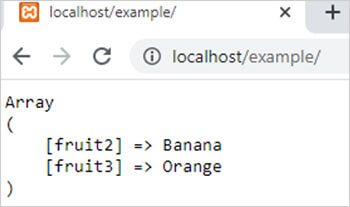array_intersect() function in php# array_intersect() function in php

1798

array_intersect() function of PHP is used to create a new array with the similar values of two existing arrays means it compare the values of two arrays and return the similar values as an array. It is an inbuilt function of PHP.

Syntax

array_intersect(\$array1,\$array2,......,\$arrayN);

Here,

\$array1,\$array2 and \$arrayN are PHP arrays.

Example:

```<?php
\$arr1= array("Apple","Banana","Orange","Pear");
\$arr2= array("Banana","Grape","Orange","Litchi");
\$result=array_intersect(\$arr1,\$arr2);
echo "<pre>";
print_r(\$result);
?>```

Output:Example 2:

array_intersect() with associative arrays return the keys of first array.

```<?php
\$arr1= array("fruit1"=>"Apple","fruit2"=>"Banana","fruit3"=>"Orange","fruit4"=>"Pear");
\$arr2= array("fruit1"=>"Banana","fruit2"=>"Grape","fruit3"=>"Orange","fruit4"=>"Litchi");
\$result=array_intersect(\$arr1,\$arr2);
echo "<pre>";
print_r(\$result);
?>```

Output:Share:## Big Hosting Days

Upto 75@ OFF on Web Hosting

## Get a .COM for just \$6.98

Secure Domain for a Mini Price